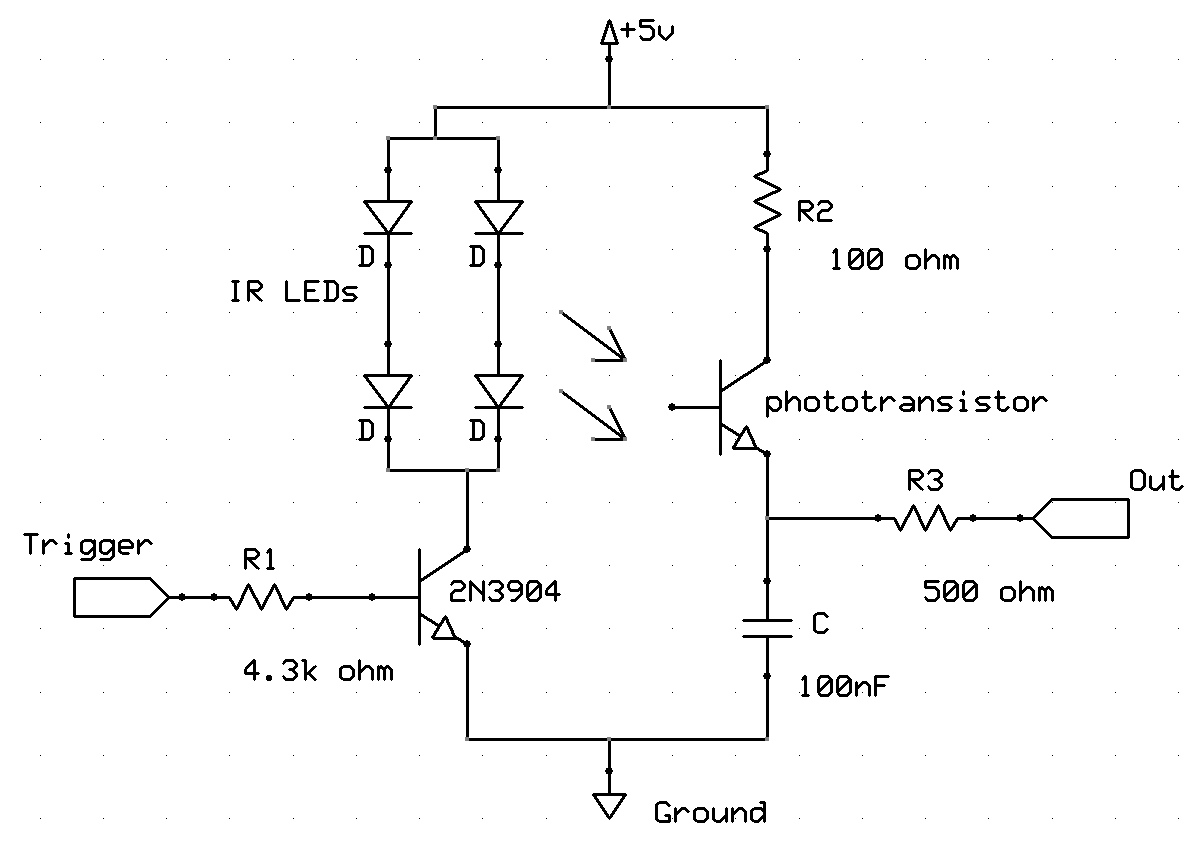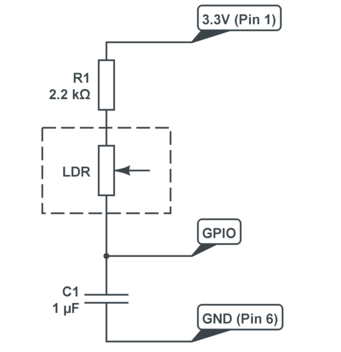## Saturday, February 8, 2014

### A simple analog proximity sensor with digital interface (for Raspberry Pi) [last update: Feb 7, 2014]

[Note: if you are using smartphone or portable device to browser this post, some math formula might not appear properly. To see the math in correct form, scroll down to the bottom and click "View web version"]

Raspberry Pi has a Broadcom BCM2835 chip, which controls 26 GPIO (general purpose input/output) pins. There are C library or RPi.GPIO python package available online that can be used to control the pins. The RPi.GPIO package is by default included in most Raspberry Pi system, such as Raspbian, a RPi version of Debian linux system.

One drawback of RPi, compared to arduino, is that it doesn't have any analog pin. All the GPIO pins are purely digital. For example, if pin A is an output pin, it can only output LOW (0V) or HIGH (3.3V), represented as 0 or 1. If pin A is an input pin, for any voltage below 0.8V applied on pin A, it takes it as LOW or 0; for any voltage above 1.3V (surprisingly low actually!), it takes it as HIGH or 1 [ref: RPi GPIO].

In real world, however, purely 0 or 1 rarely happens. We always get information that can have continuous value in its range. For example, temperature can be 10C or 50F, or 100C or 212F. These number contains more information than simply "cold" or "hot". A distance can be 2 cm or 10 m, and it is not enough to only know "close" or "far away".

There are some methods to overcome this drawback. RPi does support SPI or I2C interface, so that we can use some external analog to digital converter (ADC) and use SPI or I2C interface to get quasi-analog signal through these ADCs, such as MCP3008, TLC549, MCP23017, etc. These chips usually cost several bucks. However, with additional commercial sensors, the whole part can cost more than $20 to$30, and it is difficult to make the system compact. For robotic project one usually need more than one sensor, and the cost can add up easily.

In fact, in many situations, it is actually possible to avoid using these external devices, and still able to get analog signals through the digital pins!

The key is to convert analog signal to time duration. Because time is always analog!

I build a simple infrared proximity sensor using several infrared LEDs, one phototransistor, one 2N3904 NPN transistor, a 100nF ceramic capacitor and several low power resistors. And I am able to get some analog reading.

Here is the circuit.Infrared Proximity Sensor
All the elements are among the cheapest in the electronic market.

It doesn't really matter what LEDs, phototransistor or NPN transistors are being used. They are pretty much the same.

The only thing that might matter a little bit is the 100nF (0.1uF) capacitor. I used a low profile ceramic one, which is probably not the best choice. A class 1 ceramic, or film capacitor, will be more suitable here.

Here is the real image of the sensor.The infrared proximity sensor. The phototransistor is protected by a black rubber cylinder and surrounded by four infrared LEDs. You can also find the ceramic capacitor (upper left) and two resistors. A third one is on the other side. The sensor has four wires, +5V, GND, Trigger and OUT. The whole sensor is about 1.5cm by 2cm.

Connect the +5V and GND wires to an external 5V power supply, also connect the GND wire to the ground of the Raspberry Pi GPIO pins. Choose one GPIO pin, say, Pin A as the trigger and connect it to the Trigger wire. Choose another GPIO pin, say, Pin B, as the signal input/output and connect it to the OUT wire.

To measure the distance of an object, we send a trigger signal to activate the infrared LEDs. The light emitted by these LEDs are then reflected by the object in front of the sensor. The phototransistor in the middle collects the reflected light and generates a proportional current. This current is used to integrate the voltage across the capacitor (I=CdV/dt). By monitoring the time it takes the capacitor voltage to reach some certain threshold, we have a sense of how much current was generated by the phototransistor, or equivalently, how much light got reflected. Apparently, closer the object is, more the reflected light is. By carefully calibrating the timing of the sensor, we should be able to get a pretty precise measurement of the distance.

Here is the detailed sequential of operations.

#### 1. Zero the capacitor

First set Pin B to be an output pin and set it to be zero.

GPIO.setup(PIN_B,GPIO.OUT)
GPIO.output(PIN_B,0)

time.sleep(0.01)

This will discharge any residual voltage on the capacitor. Note that the RC time for discharging the capacitor is t=RC=500ohm * 100nF = 50 us = 0.00005 sec. By maintaining zero volt at pin B for 200RC time, we make sure the capacitor is fully discharged (the residual voltage should be $$e^{-200}=10^{-87}$$ times the original residual voltage).

#### 2. Set Pin B as Input

Now we use Pin B as an input pin to get data from the phototransisto.

GPIO.setup(PIN,GPIO.IN)

#### 3. Light up the LEDs

It's time to turn on the infrared LEDs.

GPIO.setup(PIN_A,GPIO.OUT)
GPIO.output(PIN_A,1)

This will set the voltage of trigger pin to be 3.3V. Since the BE node of 2N3904 drops 0.7V, the voltage across R1 is 2.6V. The current through R1 is then $$I=2.6V/4.3k \Omega=0.6 mA$$. 2N3904 then amplifiers this current by ~150 times, resulting a ~ 100mA current from its collector to emitter. Each of the LEDs will conduct about 50mA for a short time period.

#### 4. Timing the duration of Pin B remaining LOW

Start to measure how long it takes the capacitor to reach RPi's threshold so Pin B becomes HIGH

counter=0
t1=time.clock()*1000
while(GPIO.input(PIN_B)==0)&(counter<1e4):
counter = counter+1
deltat=time.clock()*1000-t1

deltat is the time duration of Pin B remaining LOW. Since deltat is proportional to the reciprocal of phototransistor current (or amount of reflected light), and phototransistor current is roughly proportional to the reciprocal of the distance, deltat is roughly proportional to the distance.

deltat$$\propto \frac{1}{I}\propto \frac{1}{light}\propto distance$$

The (counter<1e4) term is to prevent the situation that it takes too long to integrate the capacitor due to extremely low phototransistor current, or equivalently, infinite distance.

#### 5. Turn off the LEDs

GPIO.output(PIN_A,1)

Before using the sensor practically, we can calibrate the sensor by establishing a table of 1-to-1 correspondence of deltat and distance. When using it, after getting deltat, we just need to check the 1-to-1 table obtained during the calibration.

Now there is a clear flaw of the sensor. The amount of reflected light is not only affected by the distance, but also affected by the reflectivity of the object. A shinny mirror will definitely reflect more light compare to a sponge. I don't know if there is a simple solution to this issue. If we know what object the sensor might encounter, we can use that object to calibrate the sensor.

#### Speed

What's the speed of the sensor? It has something to do with the distance of the object, and the capacitance of C. A shorter distance or smaller C will give a faster measurement speed. I did some test with the configuration shown in the circuit above, it generally takes no more than 0.05 sec to measure distance from 0 to 10 cm (4in).

#### Minimize the affect of ambient light

Another potential issue is the ambient light. If the ambient light is too strong, it will interference with the LED light and result in an unexpected large phototransistor current, and a shorter deltat. The sensor might think it is getting too close to an object, but in fact it is facing some bright light source.

If the ambient light is fast varying, there isn't too much we can do about it, unfortunately. However, if the ambient light level remains roughly constant when measuring the distance, there is a simple solution to this issue. Since it takes about 0.05 sec to measure a distance, this requires the ambient light stays constant during this time period. Good enough for most everyday usage.

We can first perform the above steps and get a deltat , denoted as $$\delta t_1$$. Then keep the LEDs off, and redo step 1, 2, 4 to get a second deltat , denoted as  $$\delta t_2$$.

$$\delta t_2$$ is the time duration it takes the ambient light to charge the capacitor.  $$\delta t_1$$ is the time duration it takes both the ambient light and LED light to charge the capacitor.

Since (1/deltat$$\propto light$$), we need to compute

$$\delta t \equiv \frac{1}{1/\delta t_1-1/\delta t_2}=\frac{\delta t_1\delta t_2}{\delta t_2-\delta t_1}$$

The ambient light effect is then removed.

Or we can use pulse-width-modulation (PWM) to precisely control the amount the light emitted by the LEDs, and measure deltat several times with different LED brightness, and then perform a linear regression to get the true deltat given by pure LED light.

For example, set the LED power to be 0% (off), 25% (0.25 on duty during each PWM duty cycle), 50%, 75%, 100% (solid on), and get the corresponding  $$\delta t_1, \delta t_2, \delta t_3, \delta t_4, \delta t_5$$

Denote $$P$$ to be the power of LED, $$P=0, 0.25, 0.5, 0.75, 1$$.

If both the distance and ambient light remain constant, there should be

$$\frac{1}{\delta t}=\alpha P+\beta$$

$$\frac{1}{\delta t}$$ is proportional to the total light collected by the phototransistor. $$P$$ is proportional to the light emitted by the LEDs, $$\beta$$ is the effect of constant ambient light.

Obviously, coeffcient $$\alpha$$ is determined purely by the distance. In fact, $$\frac{1}{\alpha}$$ is the true deltat without any ambient light. Roughly speaking, $$\alpha$$ is proportional to 1/distance.

We perform a linear regression to $$(P_i, 1/\delta t_i)$$, where $$i=1,2,3,4,5$$. The best fit coefficient $$\alpha$$ is given by

$$\alpha \equiv \frac{<P_i/\delta t_i>-<P_i><1/\delta t_i>}{<P_i^2>-<P_i>^2}$$

where $$<x_i>\equiv\sum(x_i)/N$$ is the mean of all $$x_i$$.

Other thought

I got this idea from this post# Reading analog values from Digital Pins of Raspberry Pi

The idea of converting analog signal to time duration is very neat. The original post claims

The above technique will only work with sensors that act like resistors like photocells, thermistors, flex sensors, force-sensitive resistors, etc.

It cannot be used with sensors that have a pure analog output like IR distance sensors or analog accelerometers.

However we show that it is actually doable with devices like photodiode or so.

I am  putting the python codes into a mature package now. I will keep updating this post and include the package.

1.2.